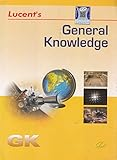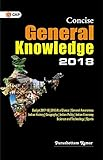# Units & Dimensions – Notes – 2

Dimentions

The exponent of a base quantity that enters into the expression, is called the dimension of the quantity in that base.

When a quantity is expressed in terms of the base quantities, it is written as a product of different powers of the base quantities.

Force is equal to mass times acceleration. Acceleration is change in velocity divided by time interval. Velocity is length divided by time interval. Thus,

Force = mass x acceleration
Force = mass x (velocity/time)
Force = mass x {(length/time)/time}
Force = mass x length x (time)-2

Thus, the dimensions of force are 1 in mass, 1 in length and – 2 in time.

Dimensional formula of some derived quantities :

 Physical quantity Expression Dimensional formula Area length × breadth [L2] Density mass / volume [ML−3] Acceleration velocity / time [LT−2] Momentum mass × velocity [MLT−1] Force mass × acceleration [MLT−2] Work force × distance [ML2T−2] Power work / time [M L2T−3] Energy work [M L2T−2] Impulse force × time [MLT−1] Radius of gyration distance [L] Pressure force / area [ML−1T−2] Surface tension force / length [MT−2] Frequency 1 / time period [T−1] Tension force [MLT−2] Moment of force (or torque) force × distance [ML2T−2] Angular velocity angular displacement / time [T−1] Stress force / area [ML−1T−2] Heat energy [ML2T−2] Heat capacity heat energy/ temperature [ML2T-2K-1] Charge current × time [AT] Faraday constant Avogadro constant ×  elementary charge [AT mol-1] Magnetic induction force / (current × length) [MT-2A-1]

 Units & Dimensions – Mcq Units & Dimensions – Notes Units & Dimensions – IQ

General Knowledge Books##-17% General Knowledge 2019 Price: INR 25.00 Was: INR 30.00-17% General Knowledge Price: INR 179.00 Was: INR 216.00-43% Objective General Knowledge Chapterwise Collection of 6250+ Questions Price: INR 169.00 Was: INR 295.00Concise General Knowledge 2018 Price: INR 30.00General Knowledge 2018 (Old Edition) Price: Check on Amazon-23% Objective General Knowledge Price: INR 302.00 Was: INR 390.00 ‹›

Be a Part of the New & Next

Share the Knowledg

Copy Protected by Chetan's WP-Copyprotect.